Previous Page | Next Page

 Language Reference

finds the univariate (scaled) median absolute deviation

MAD( (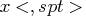))

where

x
is an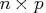input data matrix.
spt
is an optional string argument with the following values:
for computing the MAD (which is the default)
for computing the normalized version of MAD
"SN"
for computing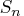"QN"
for computing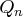The MAD function treats the input matrix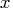as univariate data by appending each row to the previous row to make a single row vector with elements
. In the following description, the notation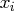means the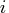th element ofwhen thought of as a row vector.

The MAD function can be used for computing one of the following three robust scale estimates:
where
is the unscaled default and
is used for the scaled version (consistency with the Gaussian distribution).
•, which is a more efficient alternative to MAD: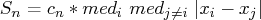where the outer median is a low median (order statistic of rank
) and the inner median is a high median (order statistic of rank
), and where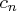is a scalar depending on sample size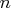.
•is another efficient alternative to MAD. It is based on the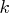th-order statistic of the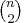inter-point distances: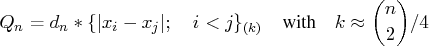where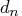is a scalar similar to but different from. See Rousseeuw and Croux (1993) for more details.
The scalarsandare defined as follows: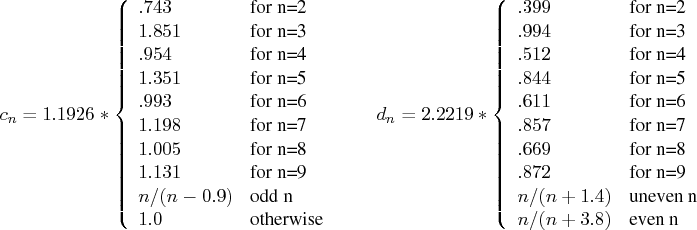### Example

The following example uses the univariate data set of Barnett and Lewis (1978). The data set is used in Chapter 9 to illustrate the univariate LMS and LTS estimates. Here is the code:


b = { 3, 4, 7, 8, 10, 949, 951 };



This program produces the following output: